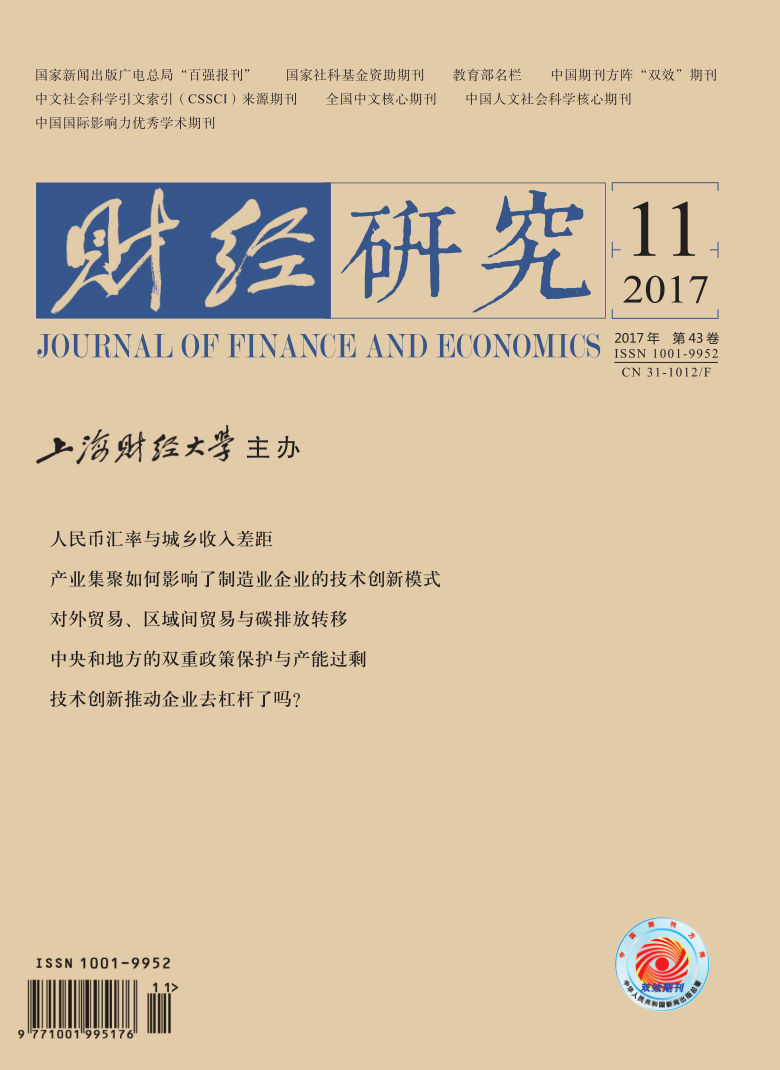﻿ 人民币汇率与城乡收入差距
 财经研究2017, Vol. 43Issue (11): 4-160

#### 文章信息财经研究2017年43卷第11期

Li Xiaolin, Si Dengkui, Jiang Chun.

Rmb exchange rate and urban-rural income gap

Journal of Finance and Economics, 2017, 43(11): 4-16.

### 文章历史《财经研究》
2017第43卷第11期

1. 中国海洋大学 经济学院，山东 青岛 266100;
2. 青岛银行，山东 青岛 266071;
3. 武汉大学 经济与管理学院，湖北 武汉 430072

RMB Exchange Rate and Urban-rural Income Gap
Li Xiaolin1,2, Si Dengkui3, Jiang Chun3
1. School of Economics, Ocean University of China, Qingdao 266100, China;
2. Bank of Qingdao, Qingdao 266071, China;
3. School of Economics and Management, Wuhan University, Wuhan 430072, China
Key words: exchange rate    income gap    panel threshold model    distribution effect

(一) 理论分析

 ${{Y}_{i}}={{Q}_{i}}\times {{P}_{i}}$ (1)

 ${{Q}_{i}}={{A}_{i}}{{L}_{i}}^{\alpha }{{K}_{i}}^{\beta }$ (2)

 ${{Y}_{i}}=({{Q}_{Ti}},{{P}_{Ti}})+({{Q}_{NTi}},{{P}_{NTi}})$ (3)

 ${{y}_{i}}=\frac{{{Y}_{i}}}{{{N}_{i}}{{P}_{Ci}}}={{a}_{i}}\frac{{{A}_{i}}{{K}_{i}}^{\beta }{{L}_{i}}^{\alpha }}{{{N}_{i}}}\frac{{{P}_{Ti}}}{{{P}_{Ci}}}+(1-{{a}_{i}})\frac{{{A}_{i}}{{K}_{i}}^{\beta }{{L}_{i}}^{\alpha }}{{{N}_{i}}}\frac{{{P}_{NTi}}}{{{P}_{Ci}}}$ (4)

① Edwards (1989) 认为均衡实际汇率是同时实现外部和内部均衡的贸易品价格和非贸易品价格之比；同时，根据巴拉萨-萨缪尔森效应，由于贸易品满足“一价定律”，因此非贸易品相对于贸易品价格的波动能够在一定程度上解释两国实际汇率水平的波动。因此，本文也将实际汇率定义为贸易品和非贸易品的相对价格，且假定贸易品采取外币定价，即实际汇率为间接标价法。

 ${{y}_{i}}=\frac{{{A}_{i}}{{K}_{i}}^{\beta }{{L}_{i}}^{\alpha }}{{{N}_{i}}}\frac{{{P}_{NTi}}}{{{P}_{Ci}}}[{{a}_{i}}{{\gamma }_{i}}+(1-{{a}_{i}})]$ (5)

 ${{P}_{Ci}}=P_{CTi}^{{{\delta }_{i}}}\times P_{CNTi}^{1-{{\delta }_{i}}}$ (6)

 ${{P}_{Ci}}={{\psi }^{\delta i}}{{P}_{CNTi}}$ (7)

 ${{y}_{i}}=A\frac{_{i}{{K}_{i}}^{\beta }{{L}_{i}}^{\alpha }}{{{N}_{i}}}\times \frac{{{P}_{NTi}}}{{{\psi }^{\delta i}}{{P}_{CNTi}}}[{{a}_{i}}{{\gamma }_{i}}+(1-{{a}_{i}})]$ (8)

 ${{y}_{i}}=A\frac{_{i}{{K}_{i}}^{\beta }{{L}_{i}}^{\alpha }}{{{N}_{i}}}\times \frac{1}{{{\psi }^{\delta i}}}[{{a}_{i}}{{\gamma }_{i}}+(1-{{a}_{i}})]$ (9)

 $I=\frac{{{y}_{u}}}{{{y}_{r}}}=\frac{{{A}_{u}}}{{{A}_{r}}}\times \frac{{{K}_{u}}^{\beta }}{{{K}_{r}}^{\beta }}\times \frac{{{L}_{u}}^{\alpha }/{{N}_{u}}}{{{L}_{r}}^{\alpha }/{{N}_{r}}}\times {{\psi }^{\delta r-\delta u}}[\frac{{{a}_{u}}\gamma +(1-{{a}_{u}})}{{{a}_{r}}\gamma +(1-{{a}_{r}})}]$ (10)

(二) 方法介绍

 {{v}_{it}}=\left\{ \begin{align} & {{\mu }_{i}}+\theta {{h}_{it}}+{{\alpha }_{1}}{{d}_{it}}+{{\varepsilon }_{it}},{{d}_{it}}\le \gamma \\ & {{\mu }_{i}}+\theta {{h}_{it}}+{{\alpha }_{2}}{{d}_{it}}+{{\varepsilon }_{it}},{{d}_{it}}>\gamma \\ \end{align} \right. (11)

 $F=\frac{{{S}_{0}}-{{S}_{1}}({{{\hat{\gamma }}}_{1}})}{{{S}_{1}}({{{\hat{\gamma }}}_{1}})/n\left( T-1 \right)}$ (12)

 $L{{R}_{1}}({{\gamma }_{1}})=\frac{{{S}_{1}}({{\gamma }_{1}})-{{S}_{1}}({{{\hat{\gamma }}}_{1}})}{{{S}_{1}}({{{\hat{\gamma }}}_{1}})/n\left( T-1 \right)},L{{R}_{2}}({{\gamma }_{2}})=\frac{{{S}_{2}}({{\gamma }_{2}})-{{S}_{2}}({{{\hat{\gamma }}}_{2}})}{{{S}_{2}}({{{\hat{\gamma }}}_{2}})/n\left( T-1 \right)}$ (13)

 $ga{{p}_{t}}=\frac{{{W}_{ut}}}{{{W}_{t}}}\ln (\frac{{{W}_{ut}}}{{{W}_{t}}}\times \frac{{{M}_{t}}}{{{M}_{ut}}})+\frac{{{W}_{rt}}}{{{W}_{t}}}\ln (\frac{{{W}_{rt}}}{{{W}_{t}}}\times \frac{{{M}_{t}}}{{{M}_{rt}}})$ (14)

 $RR{{E}_{it}}=\prod\limits_{j}^{10}{{}}{{[{{e}_{ij,t}}CP{{I}_{it}}/CP{{I}_{ij,t}}]}^{{{w}_{ij}}}},\sum\limits_{j=1}^{10}{{}}{{w}_{ij}}=1$ (15)

 $\Delta {{y}_{i,t}}={{\xi }_{i}}+{{\delta }_{i}}y_{i,t-1}^{3}+\sum\limits_{j=1}^{k}{{}}{{\theta }_{i,j}}\Delta {{y}_{i,t-j}}+{{a}_{i,t}}\sin \left( \frac{2\pi kt}{T} \right)+{{b}_{i,t}}\cos \left( \frac{2\pi kt}{T} \right)+{{\varepsilon }_{i,t}}$ (16)

 变量 GAP RRE TO FO URR PGDP 模型设定 (C, T) (C, T) (C, T) (C, T) (C, T) (C, T) 统计量t -2.313** -4.996** -2.362** -4.773*** -2.015** -1.669* 拔靴p值 0.015 0.000 0.016 0.002 0.043 0.074 突变点个数 1 2 2 2 2 1 注：***、**和*分别表示1%、5%和10%的显著性水平。下同。

 门槛变量 假设检验 F值 P值 门槛值 临界值 10% 5% 1% TO 单一门槛 32.201* 0.090 10.343 31.511 36.249 59.896 双重门槛 18.269 0.500 14.032 34.949 42.925 47.722 FO 单一门槛 20.606 0.300 0.932 27.710 30.480 40.258 双重门槛 8.035 0.670 0.164 17.874 19.029 25.049 URR 单一门槛 12.318 0.840 6.975 43.781 49.255 57.208 双重门槛 7.493 0.930 3.514 33.024 36.474 44.509 PGDP 单一门槛 122.110*** 0.000 19265 67.486 79.098 84.924 双重门槛 25.803* 0.070 33969 22.193 26.396 31.678

1.以人均收入水平为门槛变量的第一轮分组

 人均收入水平 个数 省份 低收入水平的省份(PGDP < 19 264元) 18 河北、山西、吉林、安徽、江西、河南、湖北、湖南、广西、重庆、四川、贵州、云南、陕西、甘肃、青海、宁夏、新疆 中等收入水平的省份(19 264元≤PGDP < 33 969元) 7 内蒙古、辽宁、江苏、浙江、福建、山东、广东 高收入水平的省份(PGDP≥33 969元) 3 北京、天津、上海

2.以贸易开放度为门槛变量的第二轮分组

 贸易开放程度 个数 省份 低开放程度的省份(TO < 10.343%) 25 天津、河北、山西、内蒙古、辽宁、吉林、江苏、浙江、安徽、福建、江西、山东、河南、湖北、湖南、广西、重庆、四川、贵州、云南、陕西、甘肃、青海、宁夏、新疆 高开放程度的省份(TO≥10.343%) 3 北京、上海、广东

3.样本省份的内生分组结果

 组别 组内特征 个数 省份 1 低收入水平、低贸易开放度 18 河北、山西、吉林、安徽、江西、河南、湖北、湖南、广西、重庆、四川、贵州、云南、陕西、甘肃、青海、宁夏、新疆 2 中等收入水平、低贸易开放度 6 内蒙古、辽宁、浙江、江苏、福建、山东 3 中等收入水平、高贸易开放度 1 广东 4 高收入水平、低贸易开放度 1 天津 5 高收入水平、高贸易开放度 2 北京、上海

 解释变量 组1 组2 组3 组4 组5 RRE -0.001*** 0.0004 -0.002*** 0.0002* 0.0007* TO -0.029*** 0.009*** -0.0003* 0.002* 0.002** FO 0.036* -0.050*** -0.018** -0.012* -0.012* URR 0.010*** 0.006*** 0.002* 0.0001* 0.002** GDP 0.0003 0.0005*** -0.004 -0.053 -0.009*** 常数项 0.147 0.024 0.316 0.061* -0.002 组间R2 0.419 0.459 0.798 0.745 0.524 样本数 360 120 20 20 40 注：***、**和*分别表示1%、5%和10%的显著性水平。

  钞小静, 沈坤荣. 城乡收入差距、劳动力质量与中国经济增长[J]. 经济研究, 2014(6): 30–43.  陈斌开, 林毅夫. 发展战略、城市化与中国城乡收入差距[J]. 中国社会科学, 2013(4): 81–102.  郭桂霞, 彭艳. 我国资本账户开放的门槛效应研究[J]. 金融研究, 2016(3): 42–58.  江春, 司登奎, 苏志伟. 中国城乡收入差距的动态变化及影响因素研究[J]. 数量经济技术经济研究, 2016(2): 41–57.  李实, 罗楚亮. 中国收入差距究竟有多大?——对修正样本结构偏差的尝试[J]. 经济研究, 2011(4): 68–79.  欧阳志刚. 中国城乡经济一体化的推进是否阻滞了城乡收入差距的扩大[J]. 世界经济, 2014(2): 116–135.  司登奎. 汇率预期对资产价格的非线性影响研究[D]. 青岛: 中国海洋大学, 2015. http://cdmd.cnki.com.cn/Article/CDMD-10423-1015714864.htm  王少平, 欧阳志刚. 中国城乡收入差距对实际经济增长的阈值效应[J]. 中国社会科学, 2008(2): 54–66.  张琳, 廉永辉. 实际汇率、产业结构和城乡收入差距[J]. 西安交通大学学报(社会科学版), 2013(6): 7–15.  赵永平, 徐盈之. 新型城镇化对缩小城乡收入差距的作用——基于城乡二元收入的理论模型与实证检验[J]. 中南大学学报(社会科学版), 2014(4): 37–42.  Álvarez R, López R A. Skill upgrading and the real exchange rate[J]. The World Economy, 2009, 32(8): 1165–1179. DOI:10.1111/twec.2009.32.issue-8  Bourguignon F, De Melo J, Morrisson C. Poverty and income distribution during adjustment:Issues and evidence from the OECD project[J]. World Development, 1991, 19(11): 1485–1508. DOI:10.1016/0305-750X(91)90002-Y  Chortareas G, Kapetanios G. Getting PPP right:Identifying mean-reverting real exchange rates in panels[J]. Journal of Banking & Finance, 2009, 33(2): 390–404.  Hansen B E. Threshold effects in non-dynamic panels:Estimation, testing, and inference[J]. Journal of Econometrics, 1999, 93(2): 345–368. DOI:10.1016/S0304-4076(99)00025-1  Jaumotte F, Lall S, Papageorgiou C. Rising income inequality:Technology, or trade and financial globalization?[J]. IMF Economic Review, 2013, 61(2): 271–309. DOI:10.1057/imfer.2013.7  Jeanneney S G, Hua P. How does real exchange rate influence income inequality between urban and rural areas in China?[J]. Journal of Development Economics, 2001, 64(2): 529–545. DOI:10.1016/S0304-3878(00)00149-8  Minot N W. Distributional and nutritional impact of devaluation in Rwanda[J]. Economic Development and Cultural Change, 1998, 46(2): 379–402. DOI:10.1086/452343  Theil H. Economics and information theory[M]. Amsterdam: North-Holland, 1967.